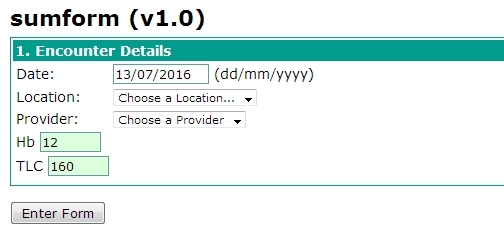# Doing calculations/logic in HTML form

Hello friends, i have started experimenting with creating custom forms via html form entry module.I have limited java script/j query knowledge. Could you help me as to why thi code is not working. Actually i have three variables(concept ID) pre configured in dictionary ( Hb,TLC and sum( which is to be a sum of hb and TLC). I wish once i enter Hb and TLC values sum(which would be a some of Hb and TLC autofill itself ) before submitting form so that i can have , three indicators.Hb,TLC and sumform (which is to be derived and is a sum of Hb and TLC Here is some of non working code i have tried so far…I unserstand it is an html java script question ,but i would greatly appreciate if you helped me get past it workin code .follows…

``````
<tr>
<td><obs conceptId="162828" labelText="Hb"/></td>
</tr>

<tr>
<td><obs conceptId="162829" labelText="TLC"/></td>
</tr>
<tr>
<td><button onclick="calculateAandB()">Sum it</button></td></tr>

<tr>
<td><span Id="calculated-value"></span></td></tr>

<script>
function calculateAandB() {
var displayVal = getValue("162828") + getValue("162829");
\$j('#calculated-value').html(displayVal);
}

getField("162828").change(calculateAandB);
getField("162829").change(calculateAandB);
</script>

`````````

Other unsuccesful approach i took…``````<tr>
<td><obs conceptId="162828" labelText="Hb"/></td>
</tr>

<tr>
<td><obs conceptId="162829" labelText="TLC"/></td>
</tr>
<tr>
<td><button onclick="calculateAandB()">Sum it</button></td></tr>

<tr>
<td><span Id="calculated-value"></span></td></tr>

<script>
function calculateAandB() {
var displayVal = getValue("162828") + getValue("162829");
\$j('#calculated-value').html(displayVal);
}

getField("162828").change(calculateAandB);
getField("162829").change(calculateAandB);
</script>

`````````

@anupam you need to put an “id” attribute on the obs tag, and then you need to refer to “yourid.value” in the getValue and getField functions.

See the link that Daniel shared in the post before this one.

@darius I improvised code like this…

``````<tr>
<td><obs conceptId="162826" labelText="Hb"/></td>
</tr>

<tr>
<td><obs conceptId="162827"

labelText="TLC"/></td>
</tr>
<tr>
<td><button onclick="calculateAandB()">Sum it</button></td></tr>

<tr>
<td><span Id="162828" labelText="Sum"></span></td></tr>

<script>
function calculateAandB() {
var displayVal = getValue("162826") + getValue("1628227");
\$j('#162828').html(displayVal);
}

getField("162826").change(calculateAandB);
getField("162827").change(calculateAandB);

</script>
```
``````<obs conceptId="..." id="tlc"/>